## The formula for compound interest is: Where: A = the future value of the investment (including interest) P = t

Question

The formula for compound interest is:

Where:

A = the future value of the investment (including interest)
P = the principal investment amount (the initial deposit amount)
r = the annual interest rate (decimal)
n = the number of times that interest is compounded per year
t = the number of years the money is invested for

If Josh invests $500 in a 5-year fixed interest savings bond that pays 5% per annum, how much will his entire investment be worth at the end of the term? in progress 0 2 months 2021-07-30T15:50:21+00:00 1 Answers 14 views 0 ## Answers ( ) 1. Answer: Future value, A =$642

Step-by-step explanation:

Given the following data;

Principal = $500 Interest rate = 5% = 5/100 = 0.05 Time, t = 5 years n = 365 To find the future value, we would use the compound interest formula;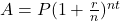Where; A is the future value. P is the principal or starting amount. r is annual interest rate. n is the number of times the interest is compounded in a year. t is the number of years for the compound interest. Substituting into the equation, we have;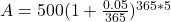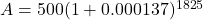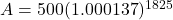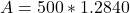Future value, A =$642